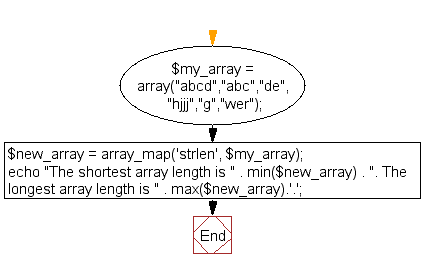﻿ PHP Array Exercise: Get the shortest, longest string length from an array - w3resource# PHP Array Exercises : Get the shortest/longest string length from an array

## PHP Array: Exercise-14 with Solution

Write a PHP script to get the shortest/longest string length from an array.

Sample arrays : ("abcd","abc","de","hjjj","g","wer")

Sample Solution:

PHP Code:

``````<?php
\$my_array = array("abcd","abc","de","hjjj","g","wer");
\$new_array = array_map('strlen', \$my_array);
// Show maximum and minimum string length using max() function and min() function
echo "The shortest array length is " . min(\$new_array) .
". The longest array length is " . max(\$new_array).'.';
?>
```
```

Sample Output:

```The shortest array length is 1. The longest array length is 4.
```

Flowchart:PHP Code Editor:

What is the difficulty level of this exercise?

﻿

## PHP: Tips of the Day

PHP: Extract numbers from a string

```\$str = 'In My Cart : 11 12 items';
preg_match_all('!\d+!', \$str, \$matches);
print_r(\$matches);
```